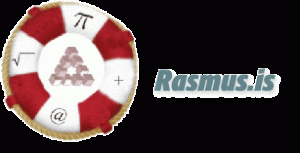Main subject: Math
related subject: Math
Duration: 30 minutes.
Age groups: Age 12
Submitted by: Lísbet Patrisía Gísladóttir. Iceland.

### Learning objectives:

Learning objectives are: Lesson 3 in Fractions. The students review/learn how to make two fractions equal, to reduce and simplify fractions, to multiply fractions and writing fractions as decimals.

### Implemented digital tools:

Device with internet connection. One for the teacher and one for each student or group. Digital tool is a website, http://rasmus.is/

### Supported digital competence for student:

Communication and collaboration, Problem solving

### Elaboration of the competences:

To find out if the students understand the material that has been teached and review the material.

### Preparation:

The students gets their digital devices and go to the webside, http://rasmus.is/ and choose the language the teacher want them to work with. Then they press start and choose grade primary. The teacher can print out ,,Your Checklist” for each student to write down the results. The students are going to work in pairs so the teacher has divided the class into pairs.

### introduction

The students choose Fractions and open Lesson 3.

### Implementation:

When the students have open Lesson 3 they read the introduction about: How to make two fractions equal, how to reduce and simplify fractions, how to multiply fractions and writing fractions as decimals. When they are finished the students choose Quiz 3 and send their answers to get their results.

### Conclusion:

The students review their results, write it down and show it to the teacher. If there are some incorrect answers the students try to solve them with their teacher.